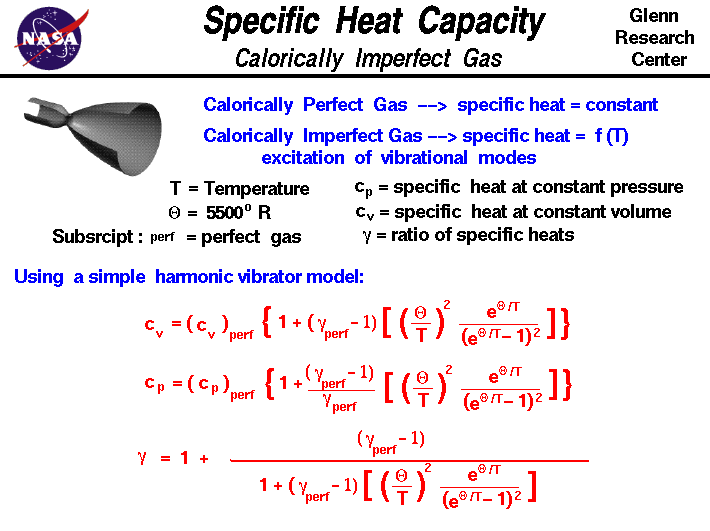+ Text Only Site
+ Non-Flash Version
+ Contact GlennThermodynamics is a branch of physics which deals with the energy and work of a system. Thermodynamics deals only with the large scale response of a system which we can observe and measure in experiments. In aerodynamics, we are most interested in thermodynamics for the role it plays in high speed flight and engine design. High temperature real gas effects play a major role in hypersonic aerodynamics. As the flight Mach number increases above Mach = 3, some of the kinetic energy of the moving object is converted into heat. Many fluid dynamics processes, like isentropic flows and shock waves, are altered by real gas effects. On the figure we present some equations that relate the specific heat capacities of air at hypersonic conditions to the specific heat capacities at lower speeds. For air at low speeds, the ratio of the specific heat capacities is a numerical constant equal to 1.4. If the specific heat capacity is a constant value, the gas is said to be calorically perfect and if the specific heat capacity changes with temperature, the gas is said to be calorically imperfect. At subsonic and low supersonic Mach numbers, air is calorically perfect. But under low hypersonic conditions, air is calorically imperfect. The specific heat capacity changes with the temperature of the flow because of excitation of the vibrational modes of the diatomic nitrogen and oxygen of the atmosphere. This computer simulation illustrates molecular vibration: This page shows an interactive Java applet which demonstrates the vibrational mode of a diatomic molecule. Click on the slider bar, hold the mouse button down and drag to the right to increase the temperature. As the temperature increases, the vibration increases and more energy is associated with the vibration. The equations shown on the figure were developed using the kinetic theory of gases including a simple harmonic vibrator for the diatomic gases. The details of the analysis were given by Eggars in NACA Report 959. A synopsis of the report is included in NACA Report 1135. The equation for the specific heat capacity at constant volume is: cv = (cv)perf * (1 + (gamp - 1) * [(theta/T)^2 * e^(theta/T) /(e^(theta/T) -1)^2]) where cv is the specific heat capacity at constant volume, (cv)perf is the specific heat capacity for a calorically perfect gas, gamp is the ratio of heat capacities for a perfect gas, theta is a thermal constant equal to 5500 degrees Rankine, and T is the static temperature. Similarly, for the specific heat capacity at constant pressure: cp = (cp)perf * (1 + ((gamp - 1)/gamp) * [(theta/T)^2 * e^(theta/T) /(e^(theta/T) -1)^2]) where cp is the specific heat capacity at constant pressure, and (cp)perf is the specific heat capacity for a calorically perfect gas. The ratio of specific heats is designated by gam, which is given by: gam = 1 + (gamp - 1) / ( 1 + (gamp-1) * [(theta/T)^2 * e^(theta/T) /(e^(theta/T) -1)^2]) The variation of the value of the specific heat capacities with temperature causes the values of many derived functions and processes to also become functions of temperature in hypersonic flows. Activities: Guided Tours Navigation.. Beginner's Guide Home Page+ Inspector General Hotline + Equal Employment Opportunity Data Posted Pursuant to the No Fear Act + Budgets, Strategic Plans and Accountability Reports + Freedom of Information Act + The President's Management Agenda + NASA Privacy Statement, Disclaimer, and Accessibility CertificationEditor: Tom Benson NASA Official: Tom Benson Last Updated: May 07 2021 + Contact Glenn